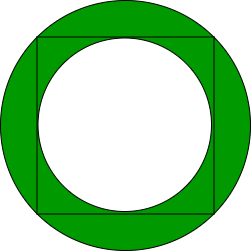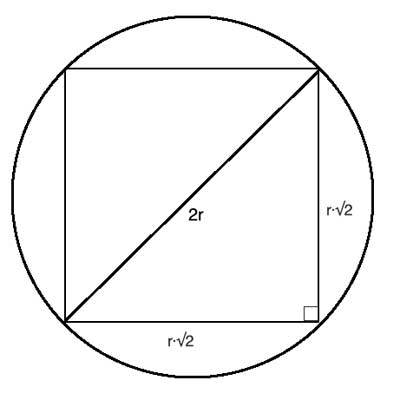# Area of the circle that has a square and a circle inscribed in it

Given the side of a square a which is kept inside a circle. It keeps expanding until all four of its vertices touch the circumference of the circle. Another smaller circle is kept inside the square now and it keeps expanding until its circumference touches all the four sides of the square. The outer and the inner circle form a ring. Find the area of this shaded part as shown in the image below.Examples:

Input: a = 3
Output: 7.06858

Input: a = 4
Output: 12.566371

## Recommended: Please try your approach on {IDE} first, before moving on to the solution.

Approach:From the above figure, R = a / sqrt(2) can be derived where a is the side length of the square. The area of the outer circle is (pi * R * R).Let s1 be the area of the outer circle (pi * R * R) and s2 be the area of the inner circle (pi * r * r). Then the area of the ring is s1 – s2.

Below is the implementation of the above approach:

## C++

 `// C++ implementation of the approach ` `#include ` `using` `namespace` `std; ` ` `  `// Function to return the required area ` `float` `getArea(``int` `a) ` `{ ` ` `  `    ``// Calculate the area ` `    ``float` `area = (M_PI * a * a) / 4.0; ` `    ``return` `area; ` `} ` ` `  `// Driver code ` `int` `main() ` `{ ` `    ``int` `a = 3; ` ` `  `    ``cout << getArea(a); ` ` `  `    ``return` `0; ` `} `

## Java

 `// Java implementation of the approach ` `class` `GFG { ` ` `  `    ``// Function to return the required area ` `    ``static` `float` `getArea(``int` `a) ` `    ``{ ` ` `  `        ``// Calculate the area ` `        ``float` `area = (``float``)(Math.PI * a * a) / ``4``; ` `        ``return` `area; ` `    ``} ` ` `  `    ``// Driver code ` `    ``public` `static` `void` `main(String args[]) ` `    ``{ ` `        ``int` `a = ``3``; ` `        ``System.out.println(getArea(a)); ` `    ``} ` `} `

## Python

 `# Python3 implementation of the approach ` `import` `math  ` ` `  `# Function to return the required area ` `def` `getArea(a): ` `     `  `    ``# Calculate the area ` `    ``area ``=` `(math.pi ``*` `a ``*` `a) ``/` `4` `    ``return` `area ` `     `  `# Driver code ` `a ``=` `3` `print``(``'{0:.6f}'``.``format``(getArea(a))) `

## C#

 `// C# implementation of the approach ` `using` `System; ` ` `  `class` `GFG  ` `{ ` ` `  `    ``// Function to return the required area ` `    ``static` `float` `getArea(``int` `a) ` `    ``{ ` ` `  `        ``// Calculate the area ` `        ``float` `area = (``float``)(Math.PI * a * a) / 4; ` `        ``return` `area; ` `    ``} ` ` `  `    ``// Driver code ` `    ``public` `static` `void` `Main() ` `    ``{ ` `        ``int` `a = 3; ` `        ``Console.Write(getArea(a)); ` `    ``} ` `} ` ` `  `// This code is contributed by mohit kumar 29 `

Output:

```7.06858
```

Don’t stop now and take your learning to the next level. Learn all the important concepts of Data Structures and Algorithms with the help of the most trusted course: DSA Self Paced. Become industry ready at a student-friendly price.

My Personal Notes arrow_drop_upCheck out this Author's contributed articles.

If you like GeeksforGeeks and would like to contribute, you can also write an article using contribute.geeksforgeeks.org or mail your article to contribute@geeksforgeeks.org. See your article appearing on the GeeksforGeeks main page and help other Geeks.

Please Improve this article if you find anything incorrect by clicking on the "Improve Article" button below.

Improved By : mohit kumar 29